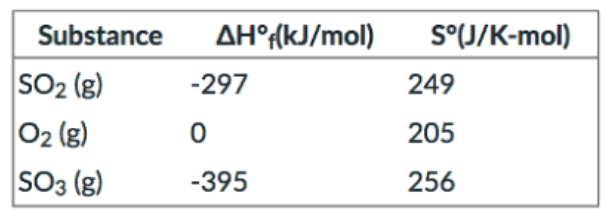# Problem: Given the thermodynamic data in the table below, calculate the equilibrium constant (at 298 K) for the reaction:2SO2 (g) + O2(g) ⇌ 2SO3(g)a) 2.37 x 1024b) 1.06c) 1.95d) 3.92 x 1023e) More information is needed.

###### FREE Expert Solution
99% (164 ratings)
###### FREE Expert Solution

We’re being asked to determine the equilibrium constant (K) at 298 K for the given reaction:

2 SO2(g) + O2(g)  2 SO3(g)

Recall that ΔG˚rxn and K are related to each other:

$\overline{){\mathbf{\Delta G}}{{\mathbf{°}}}_{{\mathbf{rxn}}}{\mathbf{=}}{\mathbf{-}}{\mathbf{RTlnK}}}$

We’re given the ΔH˚f and S˚ of each reactant and product:

 Substance ΔH˚f (kJ/mol) S˚ (J/mol • K) SO2(g) –297 249 O2(g) 0 205 SO3(g) –395 256

We can use the following equation to solve for ΔG˚rxn:

$\overline{){\mathbf{\Delta G}}{{\mathbf{°}}}_{{\mathbf{rxn}}}{\mathbf{=}}{\mathbf{\Delta H}}{{\mathbf{°}}}_{{\mathbf{rxn}}}{\mathbf{-}}{\mathbf{T\Delta S}}{{\mathbf{°}}}_{{\mathbf{rxn}}}}$

For this problem, we need to do the following steps:

Step 1: Calculate ΔH˚rxn.

Step 2: Calculate ΔS˚rxn.

Step 3: Use ΔH˚rxn and ΔS˚rxn to calculate for ΔG˚rxn.

Step 4: Calculate for K.

99% (164 ratings)###### Problem Details

Given the thermodynamic data in the table below, calculate the equilibrium constant (at 298 K) for the reaction:

2SO2 (g) + O2(g) ⇌ 2SO3(g)a) 2.37 x 1024

b) 1.06

c) 1.95

d) 3.92 x 1023

What scientific concept do you need to know in order to solve this problem?

Our tutors have indicated that to solve this problem you will need to apply the Gibbs Free Energy concept. You can view video lessons to learn Gibbs Free Energy. Or if you need more Gibbs Free Energy practice, you can also practice Gibbs Free Energy practice problems.

What is the difficulty of this problem?

Our tutors rated the difficulty ofGiven the thermodynamic data in the table below, calculate t...as high difficulty.

How long does this problem take to solve?

Our expert Chemistry tutor, Dasha took 17 minutes and 39 seconds to solve this problem. You can follow their steps in the video explanation above.

What professor is this problem relevant for?

Based on our data, we think this problem is relevant for Professor Bindell's class at UCF.